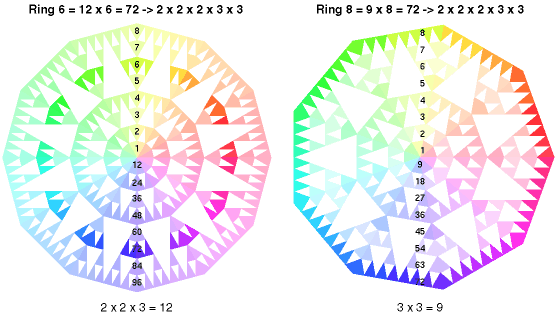Home  >   Fraktal Astrology  >   Fractal Stars - Base Geometry

 Fractal Stars - Base Geometry The base geometry of the Fractal Stars determines the hierarchy of divisions being shown, and in which ring (radius). Star - Comparison of base geometriesComparison of the geometries 12 (2 x 2 x 3) and 9 (3 x 3) The left star shows a resonance with a base geometry of 12 = 2x2x3. The right side shows the same resonance with a base geometry of 9 = 3x3. On the left we see the resonance in the 6th ring of the star, being a division of 12x6 = 72. The same to the right in the 8th ring of the star, being a division of 9x8 = 72. The scale shows the ring number (upper half) as well as the division (lower half). The prime number factoring of 72 results in 2 x 2 x 2 x 3 x 3 = 72. The base geometry of 12 integrates more resonances as 9, so the resonance is displayed in a ring further to the center of the star. Moreover geometries like 2x3 and 2x3x3 or other combinations of 2x2x2x3x3 would be displayed as resonant within this star geometry.

© Cenon GmbH - the sense of motion